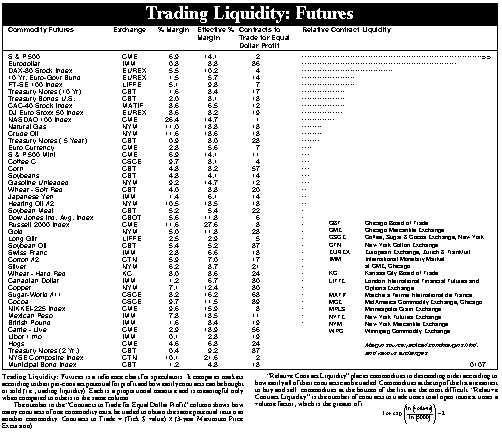# FUTURES LIQUIDITY

Trading liquidity is often overlooked as a key technical measurement in the analysis and selection of commodity futures. The following explains how to read the futures liquidity chart published by Technical Analysis of STOCKS & COMMODITIES every month. futures. The following explains how to read the futures liquidity chart published by Technical Analysis of Stocks & Commodities every month.

COMMODITY FUTURES

The futures liquidity chart shown below is intended to rank publicly traded futures contracts in order of liquidity. Relative contract liquidity is indicated by the number of dots on the right-hand side of the chart.

This liquidity ranking is produced by multiplying contract point value times the maximum conceivable price motion (based on the past three years' historical data) times the contract's open interest times a factor (usually 1 to 4) for low or very high volumes. The greatest number of dots indicates the greatest activity; futures with one or no dots show little activity and are therefore less desirable for speculators.

All futures listed are weighted equally under "contracts to trade for equal dollar profit." This is done by multiplying contract value times the maximum possible change in price observed in the last three-year period. Thus, all numbers in this column have an equal dollar value.

Columns indicating percent margin and effective percent margin provide a helpful comparison for traders who wish to place their margin money efficiently. The effective percent margin is determined by dividing the margin value (\$) by the three-year price range of contract dollar value, and then multiplying by one hundred.

STOCKS

Trading liquidity has a significant  effect on the change in price of a security. Theoretically,  trading activity can serve as a proxy for trading liquidity and equals the total volume for a given period expressed as a percentage of the total number of shares outstanding. This value can be thought of as the turnover rate of a firm's shares outstanding.Trading Liquidity: Futures is a reference chart for speculators. It compares markets according to their per-contract potential for profit and how easily contracts can be bought or sold (i.e., trading liquidity). Each is a proportional measure and is meaningful only when compared to others in the same column. The number in the "Contracts to Trade for Equal Dollar Profit" column shows how many contracts of one commodity must be traded to obtain the same potential return as another commodity. Contracts to Trade = (Tick \$ value) x (3-year Maximum Price Excursion). "Relative Contract Liquidity" places commodities in descending order according to how easily all of their contracts can be traded. Commodities at the top of the list are easiest to buy and sell; commodities at the bottom of the list are the most difficult. "Relative Contract Liquidity" is the number of contracts to trade times total open interest times a volume factor, which is the greater of: 1 or exp (1n (volume) / 1n (5000)) - 2.